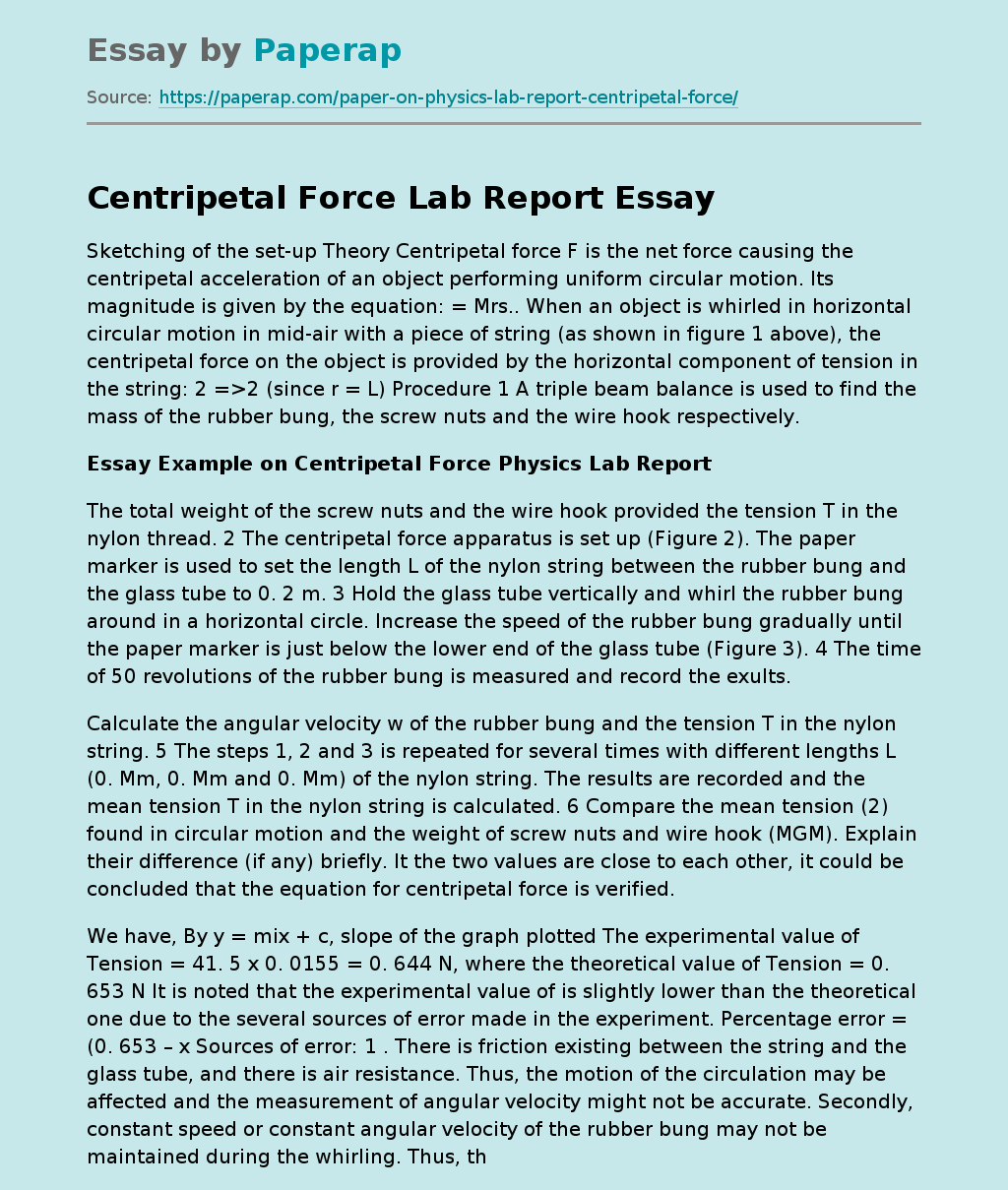# Centripetal Force Lab Report

Sketching of the set-up Theory Centripetal force F is the net force causing the centripetal acceleration of an object performing uniform circular motion. Its magnitude is given by the equation: = Mrs.. When an object is whirled in horizontal circular motion in mid-air with a piece of string (as shown in figure 1 above), the centripetal force on the object is provided by the horizontal component of tension in the string: 2 =>2 (since r = L) Procedure 1 A triple beam balance is used to find the mass of the rubber bung, the screw nuts and the wire hook respectively.

## Essay Example on Centripetal Force Physics Lab Report

The total weight of the screw nuts and the wire hook provided the tension T in the nylon thread. 2 The centripetal force apparatus is set up (Figure 2). The paper marker is used to set the length L of the nylon string between the rubber bung and the glass tube to 0. 2 m. 3 Hold the glass tube vertically and whirl the rubber bung around in a horizontal circle.

Increase the speed of the rubber bung gradually until the paper marker is just below the lower end of the glass tube (Figure 3). 4 The time of 50 revolutions of the rubber bung is measured and record the exults.

Calculate the angular velocity w of the rubber bung and the tension T in the nylon string. 5 The steps 1, 2 and 3 is repeated for several times with different lengths L (0. Mm, 0. Mm and 0. Mm) of the nylon string. The results are recorded and the mean tension T in the nylon string is calculated.

Get quality help nowwriter-CharlotteVerified

Proficient in: Force4.7 (348)

“ Amazing as always, gave her a week to finish a big assignment and came through way ahead of time. ”+84 relevant experts are online

6 Compare the mean tension (2) found in circular motion and the weight of screw nuts and wire hook (MGM). Explain their difference (if any) briefly. It the two values are close to each other, it could be concluded that the equation for centripetal force is verified.

We have, By y = mix + c, slope of the graph plotted The experimental value of Tension = 41. 5 x 0. 0155 = 0. 644 N, where the theoretical value of Tension = 0. 653 N It is noted that the experimental value of is slightly lower than the theoretical one due to the several sources of error made in the experiment. Percentage error = (0. 653 – x Sources of error: 1 . There is friction existing between the string and the glass tube, and there is air resistance. Thus, the motion of the circulation may be affected and the measurement of angular velocity might not be accurate. Secondly, constant speed or constant angular velocity of the rubber bung may not be maintained during the whirling. Thus, the measurement of angular velocity might not be accurate. 3. The radius of the circular motion and thus the length of nylon string L cannot be kept constant throughout the circular motion because there may be a variance of the force applied to keep the paper marker on the level of glass tube. Thus, the string and paper marker may move up and down and the accuracy of the tension T may be affected. 4. The stopwatch is controlled by human hands.

Thus, the reaction time of human brain (around 0. As) may lead to inaccuracy and affect the measurement of time taken and hence the accuracy of angular velocity is affected. 5. There may be miscounting in the number of circulations, because the time for 50 revolutions is quite long and students may be distracted. The accuracy of the experimental results can be improved by the following suggestions: 1. The experiment was carried out with the range of L 0. Mm, 0. Mm, 0. Mm, 0. Mm. Further experiments can be carried out beyond this range to increase the accuracy.